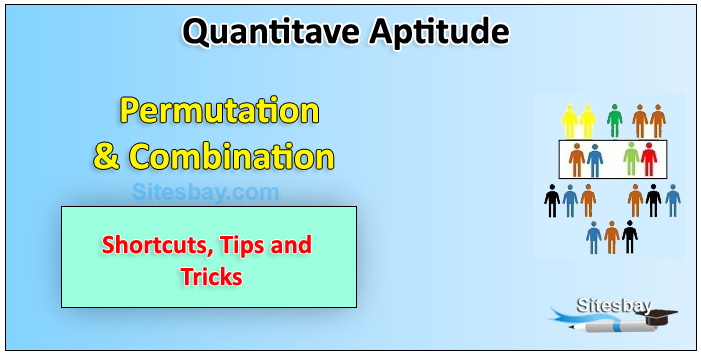# Permutation And Combination Problems

## Permutation And Combination Problems

When the order doesn't matter, it is a Combination. When the order does matter it is a Permutation. In other words A Permutation is an ordered Combination.

• Combination When the order doesn't matter, it is a Combination. A Permutation is an ordered Combination.
• Permutation When the order does matter it is a Permutation. To help you to remember, think "Permutation ... Position"The Different arrangements which can be made by taking some or all of the given things or objects at a time is called Permutation.

Each of the different selections or groups which is made by taking some or all of a number of things or objects at a time is called combination .

### Types of Permutation

There are basically two types of permutation:

• Repetition is Allowed: such as the lock above. It could be "333".
• No Repetition: for example the first three people in a running race. You can't be first and second.

### Permutations with Repetition

These are the easiest to calculate. When a thing has n different types ... we have n choices each time! For example: choosing 3 of those things, the permutations are: n * n * n (n multiplied 3 times)

More generally: choosing r of something that has n different types, the permutations are: n × n × ... (r times)

(In other words, there are n possibilities for the first choice, THEN there are n possibilities for the second choice, and so on, multiplying each time.)
Which is easier to write down using an exponent of r: n × n × ... (r times) = n r

Example: Iin the lock above, there are 10 numbers to choose from (0,1,2,3,4,5,6,7,8,9) and we choose 3 of them: 10 × 10 × ... (3 times) = 103 = 1,000 permutations

## So, the formula is simply:

```nr
where n is the number of things to choose from,
and we choose r of them,
repetition is allowed,
and order matters.
```

Question 1: In how many different ways can the letters of the word 'OPTICAL' be arranged so that the vowels always come together?

• (A) 920
• (B) 825
• (C) 720
• (D) 610

## Solution

```The word 'OPTICAL' has 7 letters. It has the vowels 'O','I','A' in it and these
3 vowels should always come together. Hence these three vowels can be
grouped and considered as a single letter. That is, PTCL(OIA).
Hence we can assume total letters as 5 and all these letters are different.
Number of ways to arrange these letters
= 5! = 5 * * 3 * 2 * 1 = 120
All the 3 vowels (OIA) are different
Number of ways to arrange these vowels among themselves
= 3! = 3* 2 * 1 = 6
Hence, required number of ways
= 120 * 6 = 720
```

Question 2: In how many different ways can the letters of the word 'CORPORATION' be arranged so that the vowels always come together?

• (A) 810
• (B) 1440
• (C) 2880
• (D) 50400

## Solution

```In the word 'CORPORATION', we treat the vowels OOAIO as one letter.

Thus, we have CRPRTN (OOAIO).

This has 7 (6 + 1) letters of which R occurs 2 times and the rest are different.
Number of ways arranging these letters = 7!/2! = 2520
Now, 5 vowels in which O occurs 3 times and the rest are different,
can be arranged in 5!/3! = 20 ways
Required number of ways = (2520 x 20) = 50400.
```

Question 3: In how many ways can the letters of the word 'LEADER' be arranged?

• (A) 72
• (B) 144
• (C) 360
• (D) 720

## Solution

```The word 'LEADER' contains 6 letters, namely 1L, 2E, 1A, 1D and 1R.
Required number of ways = 6!/(1!)(2!)(1!)(1!)(1!) = 360
```

Question 1: In how many different ways can the letters of the word 'DETAIL' be arranged in such a way that the vowels occupy only the odd positions?

• (A) 32
• (B) 48
• (C) 36
• (D) 60

## Solution

```There are 6 letters in the given word, out of which there are 3 vowels
and 3 consonants.

Let us mark these positions as under: (1) (2) (3) (4) (5) (6)

Now, 3 vowels can be placed at any of the three places out 4, marked 1, 3, 5

Number of ways of arranging the vowels = 3P3 = 3! = 6
Also, the 3 consonants can be arranged at the remaining 3 positions.

Number of ways of these arrangements = 3P3 = 3! = 6
Total number of ways = (6 x 6) = 36.
```